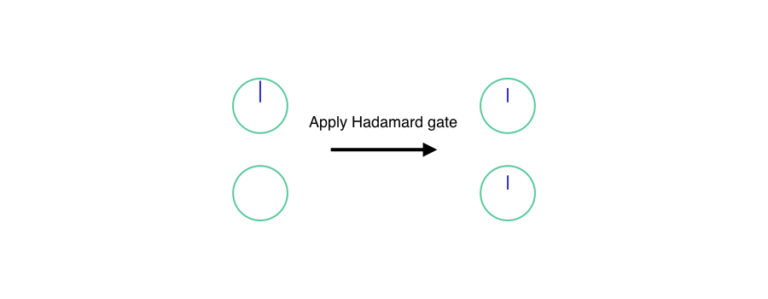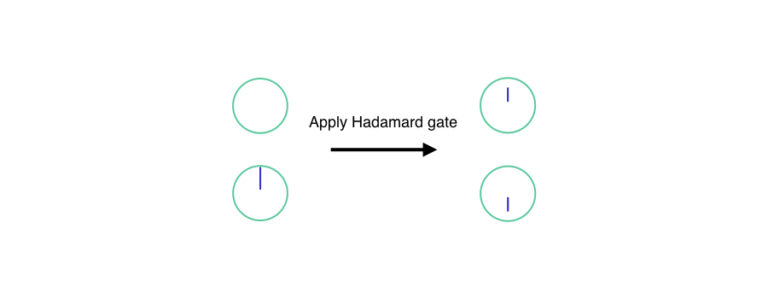•# Quantum computing: Reversible evolution

How do we design and execute quantum algorithms? Learn how quantum gates work, and why they all have to be reversible.

In quantum mechanics, every change except measurement (and noise that damages the state, known as decoherence, which we will discuss in the last week when we discuss hardware and quantum error correction) must be reversible. (In mathematical terms, this means that it can be represented by a unitary matrix.) This means that it must be possible for us to recreate the initial state using only the output state, without additional information. Here, we will introduce some of those changes as discrete operations which we call gates.

## Basic Classical Logic Gates

You may already be familiar with the most basic logic gates we use in creating a classical computer, but just in case you’re not, let’s review them. Some of the gates have one input bit and one output bit, others have two input bits and only one output bit.

### NOT

The NOT gate, as you might expect, flips a single bit.

input output
0 1
1 0

The NOT gate is reversible: if you apply a NOT gate twice to the same signal, you get out the same value you started with: NOT(NOT(X)) = X.

### AND

The AND gate takes two input bits, and its output is one only if both input bits are one:

input (A) input (B) output (C)
0 0 0
0 1 0
1 0 0
1 1 1

The AND gate is not reversible: there are four different possible input states (00, 01, 10, 11) and only two possible output states (0 and 1), so there isn’t enough information in the output to know for sure what the inputs were. In the case that the output C is 1, you know that both inputs were 1, but in all three of the other cases, you can’t tell.

### OR

The OR gate takes two input bits, and its output is zero only if both input bits are zero:

input (A) input (B) output C
0 0 0
0 1 1
1 0 1
1 1 1

Its behavior in a lot of ways is similar to that of the AND gate. The OR gate is not reversible: there are four different possible input states (00, 01, 10, 11) and only two possible output states (0 and 1), so there isn’t enough information in the output to know for sure what the inputs were. In the case that the output C is 0, you know that both inputs were 0, but in all three of the other cases, you can’t tell.

In fact, with the AND or OR gate, even if I gave you the output and one of the inputs, you wouldn’t be able to tell unambiguously what the other input value was in all cases.

### XOR

The XOR, or exclusive OR, gate takes two input bits, and its output is one only if exactly one input bit is one:

input (A) input (B) output (C)
0 0 0
0 1 1
1 0 1
1 1 0

The XOR gate is close to reversible: with two input bits and one output bit, there still isn’t enough information in the output to know for sure what the inputs were. But, if I give you one of the inputs, you can now tell unambiguously what the other input was! We will see below that one of the important reversible two-qubit gates is related to XOR.

## Reversible Classical Gates

### Entropy and Information

In the early 1970s, Charles Bennett of IBM, later joined by Richard Feynman of Caltech, and Tommaso Toffoli and Ed Fredkin of MIT, laid the groundwork for discrete reversible logic operations. Bennett was an acolyte of Rolf Landauer, who recognized that the destruction of information adds to the entropy of the Universe, and must generate waste heat.

Entropy is the amount of disorder in something. If you take a physics class, or possibly chemistry, you will learn about entropy when you learn about thermodynamics. If you have a lot of energy confined in one place, you can use it to do work, such as turning a motor. This spreads energy around. Once the energy is spread out evenly, the entropy is maximized and it is no longer possible to do useful work with it.

In computer science we also talk about the entropy of information. If you have a certain amount of data, it might be all zeroes or all ones, in which case it’s very easy to tell a friend how to recreate the same state: just say, “I have seven hundred ones.” If your data is more complex, though, it gets harder to describe; it has high entropy.

I (Van Meter) can recall taking Feynman’s class at Caltech, in which he explained the relationship between information and entropy in terms of thermodynamics. For quite a while, I believed that he was speaking allegorically, and couldn’t understand why he was bringing in physical constants. When I finally understood that he meant it quite literally, I could feel the hair on the back of my neck stand up. It remains one of the most startling ideas I have ever encountered.

### Reversible Computing

Generally, entropy increases when we lose information, or when information is erased. The AND and OR gates we discussed above necessarily lose information: they start with two input bits, and end with only one, so there is no way to carry all of the information through.

If, instead, we require all of our gates to have the same number of input and output bits, it’s possible that we might be able to undo our computation. A second additional criteria is necessary to make it reversible: every possible output state comes from exactly one input state. Then we say that the function is “one to one”, or bijective.

Any logic function can be computed using one, two, and three-bit reversible gates, so we will look at a few of them.

### Identity

The identity gate does nothing to the state: its output is the same as its input. It is obviously reversible; since it does nothing, in order to get back to where we started, we only have to do nothing one more time!

input output
0 0
1 1

### NOT

We have already seen the NOT gate above. Executing NOT twice in a row brings us back to where we started: NOT(NOT(X)) = X.

### CNOT

The controlled NOT gate, or CNOT, takes two input bits and produces two output bits. If one of the bits (called the control bit) is one, the other bit (called the target bit) is flipped. If the control bit is zero, the target bit is passed through unchanged.

input (A) input (B) output (A’) output (B’)
0 0 0 0
0 1 0 1
1 0 1 1
1 1 1 0

A quick examination of the table shows that (A’) is the same as (A), and (B’) is the XOR of (A) and (B), (B’ = A oplus B). If we apply the same gate twice, obviously (A) stays the same, and the new (B” = A oplus (A oplus B) = B), and we are back where we started.

### CCNOT (Toffoli Gate)

The control-control-NOT gate, or CCNOT gate, is also called the Toffoli gate, named for Tommaso Toffoli. It uses two control bits instead of one.

input (A) input (B) input (C) output (A’) output (B’) output (C’)
0 0 0 0 0 0
0 0 1 0 0 1
0 1 0 0 1 0
0 1 1 0 1 1
1 0 0 1 0 0
1 0 1 1 0 1
1 1 0 1 1 1
1 1 1 1 1 0

### CSWAP (Fredkin Gate)

The control-SWAP gate, or CSWAP gate, is also called the Fredkin gate, named for Ed Fredkin. If the control bit is zero, the other two bits are left alone. If the control bit is one, the other two bits are swapped.

input (A) input (B) input (C) output (A’) output (B’) output (C’)
0 0 0 0 0 0
0 0 1 0 0 1
0 1 0 0 1 0
0 1 1 0 1 1
1 0 0 1 0 0
1 0 1 1 1 0
1 1 0 1 0 1
1 1 1 1 1 1

Either the CCNOT or the CSWAP gate is powerful enough to be able to create any classical logic circuit.

## Single-Qubit Gates

The reversible gates above all have quantum equivalents, and we will use them in constructing quantum algorithms. But qubits are more complex objects than simple classical bits, allowing a broader range of operations that all qualify as unitary. Here, we will look at some single-qubit gates that go beyond just the Identity and NOT gates above.

### Rotations on the Bloch Sphere

When we introduced qubits, we introduced the notion of the Bloch sphere, and the idea of the state of a single qubit as a point on the sphere. The one-qubit gates can be understood as rotations about the X, Y, or Z axis of the Bloch sphere.

Rotation is a naturally reversible operation: just rotate back in the opposite direction by the same amount. Every point on the sphere has a starting position and an ending position, and no two points ever come together. No two different starting points come to the same ending position. This is a key factor maintaining reversibility. If two points did come together, when we attempted to reverse the operation, we would have no way of distinguishing them and returning them to separate starting positions.

### The NOT Gate and Other X Gates

A simple reversible gate, like the classical NOT gate, the (X) gate takes 0 to 1 and 1 to 0. Since our state can be a superposition, it does a little more than this, it swaps our 0 and 1 values, or the contents of our 0 and 1 dials. It is a rotation of 180 degrees, or (pi), about the (X) axis. It’s also possible to rotate by any angle, which swaps the 0 and 1 values less completely.

### The Phase Gates

Although rotating around the (X) axis is somewhat like the classical NOT gate, rotating around the (Z) axis has no classical equivalent. (Z) rotation modifies the phase of the state. We call a 180 degree rotation simply the Z gate. For other angles, we call it a Z rotation or a phase gate, and specify the angle.

The Hadamard gate is our most basic means of creating superposition. It takes our (vert0rangle) state to a 50/50 superposition of (vert0rangle) and (vert1rangle):

[vert0rangle rightarrow frac{vert0rangle + vert1rangle}{sqrt{2}}]If it also took the (vert1rangle) state to the same 50/50 superposition, it wouldn’t be reversible. Instead, it takes it to a superposition with a phase twist on the (vert1rangle) state:

[vert1rangle rightarrow frac{vert0rangle + (pi)vert1rangle}{sqrt{2}}]Applying the Hadamard twice to the same qubit returns it to its original state. In fact, this is our first use of interference! If we began with the (vert0rangle) state, we get constructive interference that builds us a new (vert0rangle) state, and destructive interference that eliminates the (vert1rangle) state:

(frac{vert0rangle + vert1rangle}{sqrt{2}}rightarrow vert0rangle).

Here we can see that the phase of the state has a critical impact on the interference; reversing the other state results in constructive interference on the (vert1rangle) state, and destructive interference on the (vert0rangle) state:

(frac{vert0rangle + (pi)vert1rangle}{sqrt{2}}rightarrow vert1rangle).